You are using an outdated browser. Please upgrade your browser to improve your experience.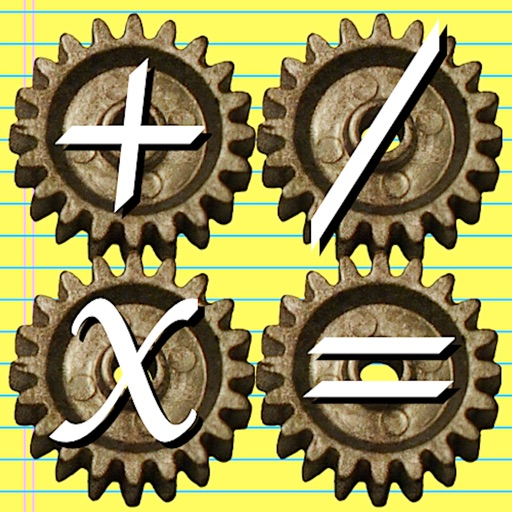## AutoMathic is a different kind of calculator, smart enough to help you solve math problems by automating as much of the work as you want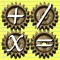# AutoMathic

by Kevin B. Belton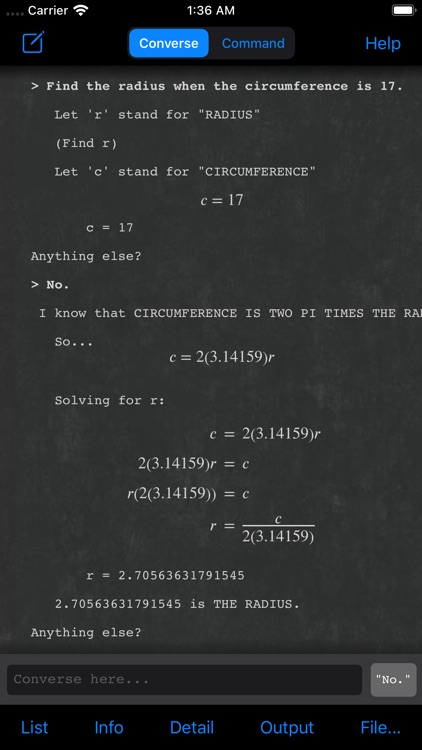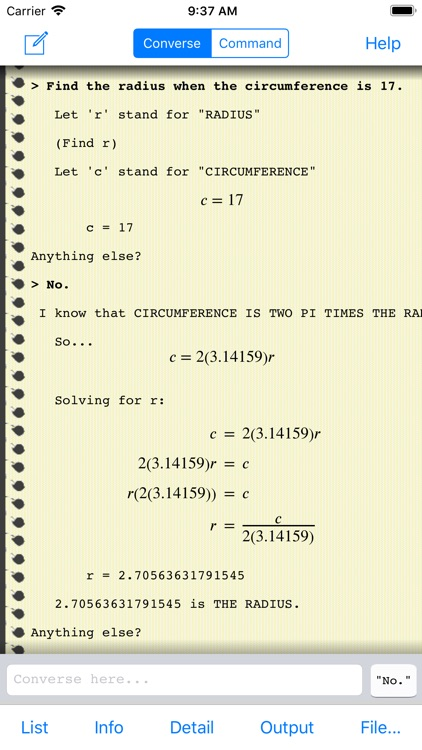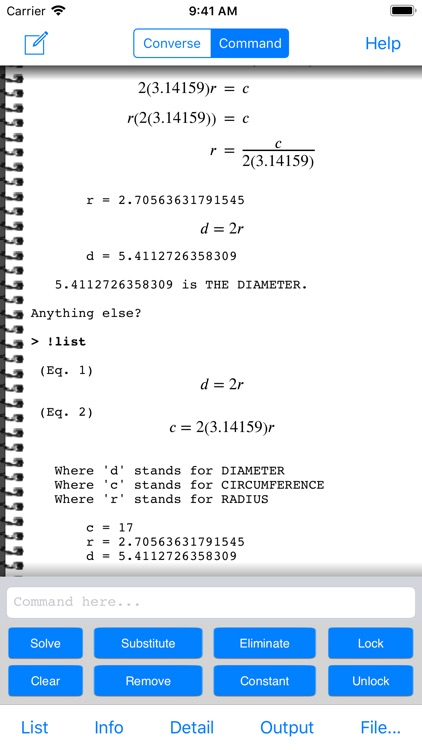### What is it about?

AutoMathic is a different kind of calculator, smart enough to help you solve math problems by automating as much of the work as you want. With AutoMathic, you focus on specifying the problem, and let the computer do the mechanical work of solving it! If your current calculator can't directly solve for input like this, you need AutoMathic:### App Details

Version
2.3.1
Rating
(2)
Size
5Mb
Genre
Utilities Education
Last updated
November 28, 2021
Release date
August 17, 2010

### App Screenshots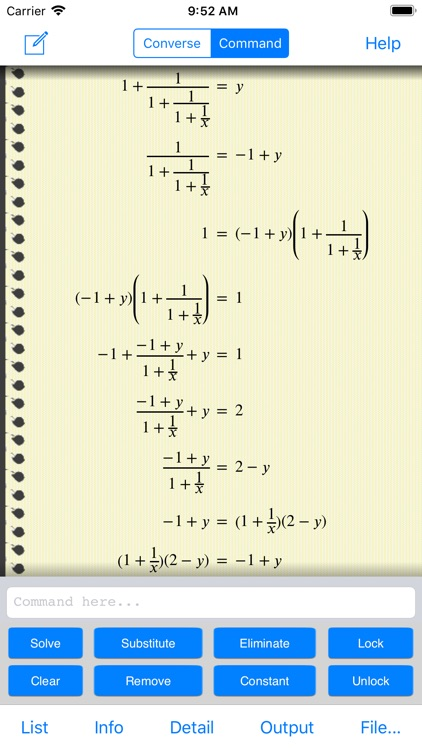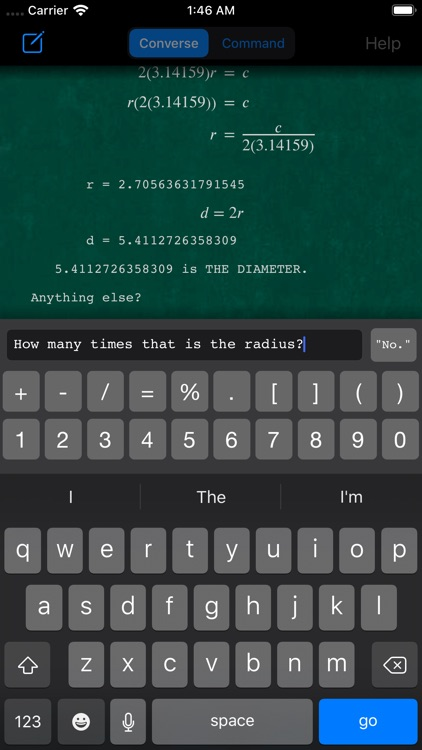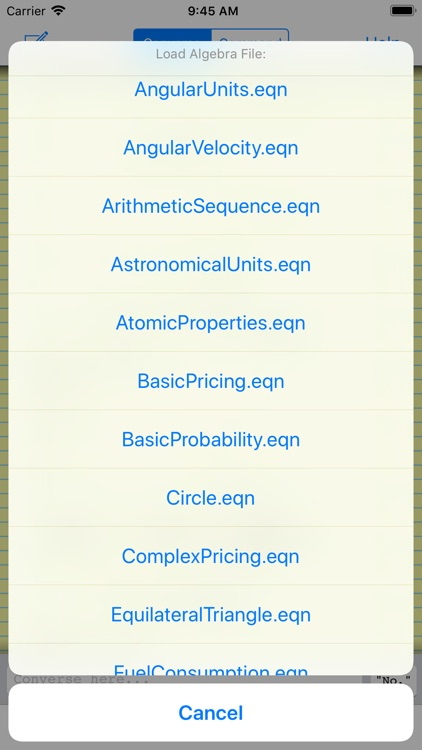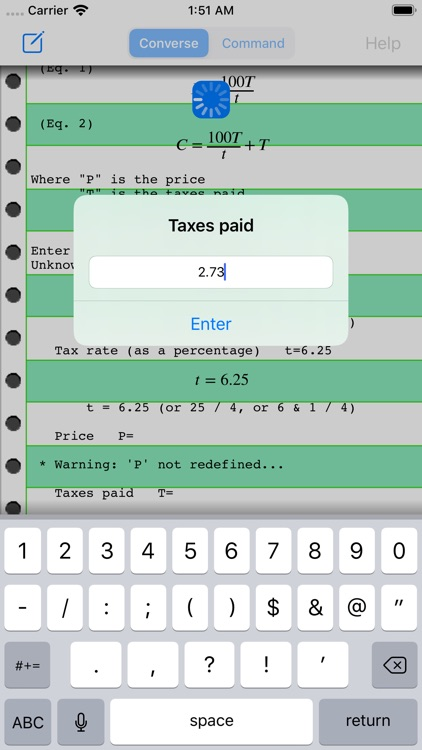### App Store Description

AutoMathic is a different kind of calculator, smart enough to help you solve math problems by automating as much of the work as you want. With AutoMathic, you focus on specifying the problem, and let the computer do the mechanical work of solving it! If your current calculator can't directly solve for input like this, you need AutoMathic:

"How many millions is 12% of 275 million?"
"What must the price have been assuming that tips were 2.75?"
"21 is the difference between what circumference and what radius?"
"How many times 2.4[mHz] is 3.1[gHz]?"
"Find the cost daily when the cost semiannually is 475."
"3[drops/sec] converts to how many [gallons/day]?"
"If the average speed is 50[mph], and the radius is 50[m], how many G's would the centripetal acceleration be?"
"If the wave speed is the speed of sound, and the frequency is F#, how many unit inches long is the wavelength?"
"How many trillionths of a unit Coulomb is the charge when the capacitance is 53[pF], and the voltage is 12[V]?"
"If the power is 40[W], and the voltage is 12[V], find the resistance measured in ohms, and the current measured in amps."
"Find the mass per particle measured in AMU when the mass for 6 moles is 84[gm]."
"If the formula weight is 106, find the number of grams such that the molar concentration is 4 when the number of ml is 1,500."
"If the density is half the density of steel, and 75% of the volume is 2[gal], find the mass measured in pounds."
"If the specific gravity is 0.668, find the density measured in lb/gal."
"Find the number of nucleons, and the atomic weight. The atomic number is 6, the ratio of protons to neutrons is 1:1, and the ionic charge is +2."
"Compute the volume measured in liters if the number of moles is 1, pressure is standard pressure, and temperature is standard temperature."
"Find the heat energy measured in Joules given that the mass is 7.05[gm], deltaT is 75, and the specific heat is the specific heat of iron."

AutoMathic is great for:
- Math and Science Students
- Teachers and Tutors
- Scientists and Engineers
- "Everyday" math
- "Off-the-cuff" calculations
- Anyone who's naturally curious or likes to play with numbers!

Calculate *your* way, with 3 complementary modes of operation:

1. AutoMathic lets you run scripted calculations from a large, built-in library if you want to do something commonplace.

2. AutoMathic lets you do manual calculations by accepting expressions and equations you enter yourself, and gives you the tools to manipulate them using familiar techniques from algebra.

3. AutoMathic lets you do free-form calculations by automatically translating your English-like, conversational input into variables, expressions, and equations to be solved... and then it solves them! You tell it what you know, and ask it what you want to find out!

Most calculators are just "calculators", not problem-solvers... AutoMathic is different:

- Instead of a one-line, numeric display, its output scrolls indefinitely, showing variables, expressions, and equations that are formatted and easy to read!

- Instead of passively waiting for directions, it automates most of the process (even when in the hands-on "Command" mode), but has customizable level-of-detail settings that can show and explain every step of the way!

- Instead of rigid equation libraries, it has a collection of task-oriented workflows that conform to how you want to use them. They don't even distinguish between "input" and "output" variables!

- Instead of requiring "programming" with a low-level programming language, it lets you create, use, save, and later reuse your own equations just like the built-in equation libraries!

- AutoMathic offers a high-level, "natural-language" interface that accepts free-form, conversational input and automatically pulls in whatever background information is needed at the time from a broad, built-in knowledgebase!

- Transcripts can be saved to record and document the entire session!

Disclaimer:
AppAdvice does not own this application and only provides images and links contained in the iTunes Search API, to help our users find the best apps to download. If you are the developer of this app and would like your information removed, please send a request to takedown@appadvice.com and your information will be removed.